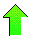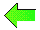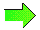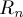Planet Quantum NewsContents News View

 Apr 20, 2010 - Challenge Problems #3-#4Hello again, friendly visitor to PLANETQUANTUM.COM.    :-)     No one has solved Challenge Problem #1 or Challenge Problem #2 yet. Today, I have two new challenge problems for you. Challenge Problem #3 is defined by raising integers in The Pi = E Problem to the power of n where n is a positive integer. In Page3n.txt, I give you the solutions of the resulting system for n=2,3,4,...,99. Can you solve this problem for n=100? As far as I've calculated (above n=100), the general problem always has a solution. Challenge Problem #4 is defined with the help of RSA numbers. For brevity, let= RSA-n . Can you solve this problem? RSA numbers (e.g. RSA-1024 and RSA-2048) occur in the RSA encryption algorithm used in public key cryptography. I have put 64 similar problems with solutions here: Den12 I will give credit on my web site to the first (up to two) people to email me correct solutions to either of these two problems. Just send me your answers and be the first if you want Fame on my web site.    :-) TXT Versions: Challenge Problem #3 and Challenge Problem #4.